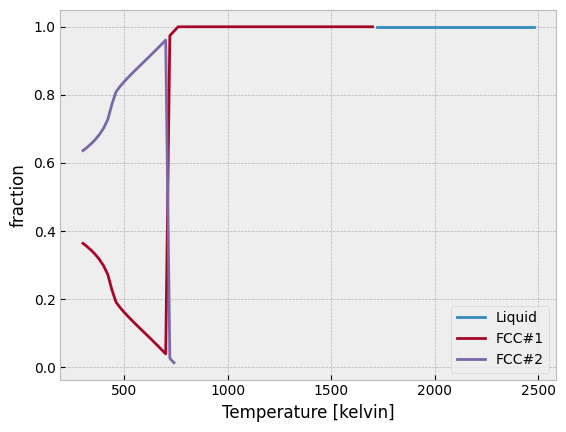# Equilibrium Properties and Partial Ordering (Al-Fe and Al-Ni)¶

:

# Only needed in a Jupyter Notebook
%matplotlib inline
# Optional plot styling
import matplotlib
matplotlib.style.use('bmh')

:

import matplotlib.pyplot as plt
import numpy as np


## Al-Fe (Heat Capacity and Degree of Ordering)¶

Here we compute equilibrium thermodynamic properties in the Al-Fe system. We know that only B2 and liquid are stable in the temperature range of interest, but we just as easily could have included all the phases in the calculation using my_phases = list(db.phases.keys()). Notice that the syntax for specifying a range is (min, max, step). We can also directly specify a list of temperatures using the list syntax, e.g., [300, 400, 500, 1400].

We explicitly indicate that we want to compute equilibrium values of the heat_capacity and degree_of_ordering properties. These are both defined in the default Model class. For a complete list, see the documentation. equilibrium will always return the Gibbs energy, chemical potentials, phase fractions and site fractions, regardless of the value of output.

:

db = Database('alfe_sei.TDB')
my_phases = ['LIQUID', 'B2_BCC']
eq = equilibrium(db, ['AL', 'FE', 'VA'], my_phases, {v.X('AL'): 0.25, v.T: (300, 2000, 50), v.P: 101325},
output=['heat_capacity', 'degree_of_ordering'])
print(eq)

<xarray.Dataset>
Dimensions:             (N: 1, P: 1, T: 34, X_AL: 1, vertex: 3, component: 2, internal_dof: 5)
Coordinates:
* N                   (N) float64 1.0
* P                   (P) float64 1.013e+05
* T                   (T) float64 300.0 350.0 400.0 ... 1.9e+03 1.95e+03
* X_AL                (X_AL) float64 0.25
* vertex              (vertex) int32 0 1 2
* component           (component) <U2 'AL' 'FE'
Dimensions without coordinates: internal_dof
Data variables:
NP                  (N, P, T, X_AL, vertex) float64 1.0 nan nan ... nan nan
GM                  (N, P, T, X_AL) float64 -2.858e+04 ... -1.413e+05
MU                  (N, P, T, X_AL, component) float64 -7.274e+04 ... -1....
X                   (N, P, T, X_AL, vertex, component) float64 0.25 ... nan
Y                   (N, P, T, X_AL, vertex, internal_dof) float64 0.5 ......
Phase               (N, P, T, X_AL, vertex) <U6 'B2_BCC' '' '' ... '' ''
degree_of_ordering  (N, P, T, X_AL, vertex) float64 0.6666 nan ... nan nan
heat_capacity       (N, P, T, X_AL) float64 25.45 26.93 ... 42.44 42.44
Attributes:
created:  2022-02-19T19:18:13.281597


We also compute degree of ordering at fixed temperature as a function of composition.

:

eq2 = equilibrium(db, ['AL', 'FE', 'VA'], 'B2_BCC', {v.X('AL'): (0,1,0.01), v.T: 700, v.P: 101325},
output='degree_of_ordering')
print(eq2)

<xarray.Dataset>
Dimensions:             (N: 1, P: 1, T: 1, X_AL: 100, vertex: 3, component: 2, internal_dof: 5)
Coordinates:
* N                   (N) float64 1.0
* P                   (P) float64 1.013e+05
* T                   (T) float64 700.0
* X_AL                (X_AL) float64 1e-10 0.01 0.02 0.03 ... 0.97 0.98 0.99
* vertex              (vertex) int32 0 1 2
* component           (component) <U2 'AL' 'FE'
Dimensions without coordinates: internal_dof
Data variables:
NP                  (N, P, T, X_AL, vertex) float64 1.0 nan nan ... nan nan
GM                  (N, P, T, X_AL) float64 -2.447e+04 ... -1.949e+04
MU                  (N, P, T, X_AL, component) float64 -2.443e+05 ... -1....
X                   (N, P, T, X_AL, vertex, component) float64 1e-10 ... nan
Y                   (N, P, T, X_AL, vertex, internal_dof) float64 9.807e-...
Phase               (N, P, T, X_AL, vertex) <U6 'B2_BCC' '' '' ... '' ''
degree_of_ordering  (N, P, T, X_AL, vertex) float64 0.009636 nan ... nan nan
Attributes:
created:  2022-02-19T19:18:13.601354


### Plots¶

Next we plot the degree of ordering versus temperature. We can see that the decrease in the degree of ordering is relatively steady and continuous. This is indicative of a second-order transition from partially ordered B2 to disordered bcc (A2).

:

plt.gca().set_title('Al-Fe: Degree of bcc ordering vs T [X(AL)=0.25]')
plt.gca().set_xlabel('Temperature (K)')
plt.gca().set_ylabel('Degree of ordering')
plt.gca().set_ylim((-0.1,1.1))
# Generate a list of all indices where B2 is stable
phase_indices = np.nonzero(eq.Phase.values == 'B2_BCC')
# phase_indices refers to all temperature indices
# We know this because pycalphad always returns indices in order like P, T, X's
plt.plot(np.take(eq['T'].values, phase_indices), eq['degree_of_ordering'].values[phase_indices])
plt.show()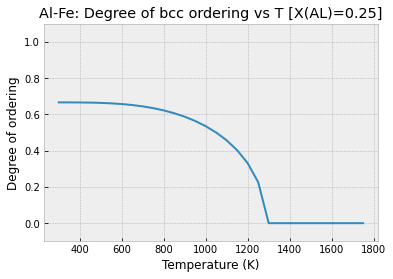For the heat capacity curve shown below we notice a sharp increase in the heat capacity around 750 K. This is indicative of a magnetic phase transition and, indeed, the temperature at the peak of the curve coincides with 75% of 1043 K, the Curie temperature of pure Fe. (Pure bcc Al is paramagnetic so it has an effective Curie temperature of 0 K.)

We also observe a sharp jump in the heat capacity near 1800 K, corresponding to the melting of the bcc phase.

:

plt.gca().set_title('Al-Fe: Heat capacity vs T [X(AL)=0.25]')
plt.gca().set_xlabel('Temperature (K)')
plt.gca().set_ylabel('Heat Capacity (J/mol-atom-K)')
# np.squeeze is used to remove all dimensions of size 1
# For a 1-D/"step" calculation, this aligns the temperature and heat capacity arrays
# In 2-D/"map" calculations, we'd have to explicitly select the composition of interest
plt.plot(eq['T'].values, np.squeeze(eq['heat_capacity'].values))
plt.show()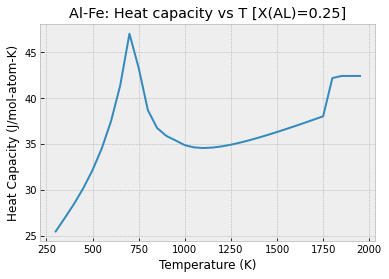To understand more about what’s happening around 700 K, we plot the degree of ordering versus composition. Note that this plot excludes all other phases except B2_BCC. We observe the presence of disordered bcc (A2) until around 13% Al or Fe, when the phase begins to order.

:

plt.gca().set_title('Al-Fe: Degree of bcc ordering vs X(AL) [T=700 K]')
plt.gca().set_xlabel('X(AL)')
plt.gca().set_ylabel('Degree of ordering')
# Select all points in the datasets where B2_BCC is stable, dropping the others
eq2_b2_bcc = eq2.where(eq2.Phase == 'B2_BCC', drop=True)
plt.plot(eq2_b2_bcc['X_AL'].values, eq2_b2_bcc['degree_of_ordering'].values.squeeze())
plt.show()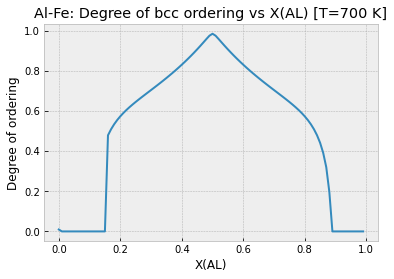## Al-Ni (Degree of Ordering)¶

:

db_alni = Database('NI_AL_DUPIN_2001.TDB')
phases = ['LIQUID', 'FCC_L12']
eq_alni = equilibrium(db_alni, ['AL', 'NI', 'VA'], phases, {v.X('AL'): 0.10, v.T: (300, 2500, 20), v.P: 101325},
output='degree_of_ordering')
print(eq_alni)

<xarray.Dataset>
Dimensions:             (N: 1, P: 1, T: 110, X_AL: 1, vertex: 3, component: 2, internal_dof: 5)
Coordinates:
* N                   (N) float64 1.0
* P                   (P) float64 1.013e+05
* T                   (T) float64 300.0 320.0 340.0 ... 2.46e+03 2.48e+03
* X_AL                (X_AL) float64 0.1
* vertex              (vertex) int32 0 1 2
* component           (component) <U2 'AL' 'NI'
Dimensions without coordinates: internal_dof
Data variables:
NP                  (N, P, T, X_AL, vertex) float64 0.3637 0.6363 ... nan
GM                  (N, P, T, X_AL) float64 -2.526e+04 ... -1.944e+05
MU                  (N, P, T, X_AL, component) float64 -1.719e+05 ... -1....
X                   (N, P, T, X_AL, vertex, component) float64 0.25 ... nan
Y                   (N, P, T, X_AL, vertex, internal_dof) float64 2.229e-...
Phase               (N, P, T, X_AL, vertex) <U7 'FCC_L12' 'FCC_L12' ... ''
degree_of_ordering  (N, P, T, X_AL, vertex) float64 1.0 6.377e-15 ... nan
Attributes:
created:  2022-02-19T19:18:16.761326


### Plots¶

In the plot below we observe two phases designated FCC_L12. This is indicative of a miscibility gap. The ordered gamma-prime phase steadily decreases in amount with increasing temperature until it completely disappears around 750 K, leaving only the disordered gamma phase.

:

from pycalphad.plot.utils import phase_legend
phase_handles, phasemap = phase_legend(phases)

plt.gca().set_title('Al-Ni: Phase fractions vs T [X(AL)=0.1]')
plt.gca().set_xlabel('Temperature (K)')
plt.gca().set_ylabel('Phase Fraction')
plt.gca().set_ylim((0,1.1))
plt.gca().set_xlim((300, 2000))

for name in phases:
phase_indices = np.nonzero(eq_alni.Phase.values == name)
plt.scatter(np.take(eq_alni['T'].values, phase_indices), eq_alni.NP.values[phase_indices], color=phasemap[name])
plt.gca().legend(phase_handles, phases, loc='lower right')

:

<matplotlib.legend.Legend at 0x1ebe70d6dc0>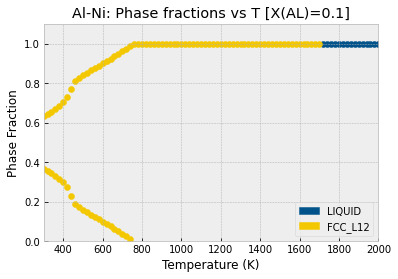In the plot below we see that the degree of ordering does not change at all in each phase. There is a very abrupt disappearance of the completely ordered gamma-prime phase, leaving the completely disordered gamma phase. This is a first-order phase transition.

:

plt.gca().set_title('Al-Ni: Degree of fcc ordering vs T [X(AL)=0.1]')
plt.gca().set_xlabel('Temperature (K)')
plt.gca().set_ylabel('Degree of ordering')
plt.gca().set_ylim((-0.1,1.1))
# Generate a list of all indices where FCC_L12 is stable and ordered
L12_phase_indices = np.nonzero(np.logical_and((eq_alni.Phase.values == 'FCC_L12'),
(eq_alni.degree_of_ordering.values > 0.01)))
# Generate a list of all indices where FCC_L12 is stable and disordered
fcc_phase_indices = np.nonzero(np.logical_and((eq_alni.Phase.values == 'FCC_L12'),
(eq_alni.degree_of_ordering.values <= 0.01)))
# phase_indices refers to all temperature indices
# We know this because pycalphad always returns indices in order like P, T, X's
plt.plot(np.take(eq_alni['T'].values, L12_phase_indices), eq_alni['degree_of_ordering'].values[L12_phase_indices],
label='$\gamma\prime$ (ordered fcc)', color='red')
plt.plot(np.take(eq_alni['T'].values, fcc_phase_indices), eq_alni['degree_of_ordering'].values[fcc_phase_indices],
label='$\gamma$ (disordered fcc)', color='blue')
plt.legend()
plt.show()

C:\Users\rotis\AppData\Local\Temp/ipykernel_7844/3149017277.py:7: RuntimeWarning: invalid value encountered in greater
(eq_alni.degree_of_ordering.values > 0.01)))
C:\Users\rotis\AppData\Local\Temp/ipykernel_7844/3149017277.py:10: RuntimeWarning: invalid value encountered in less_equal
(eq_alni.degree_of_ordering.values <= 0.01)))